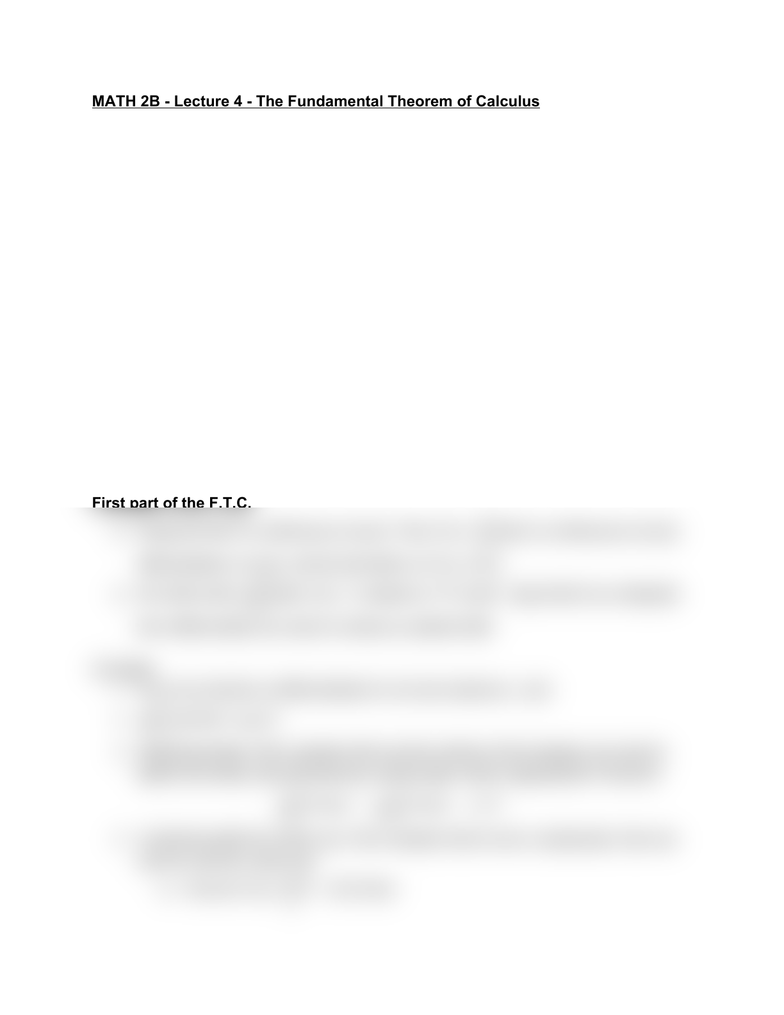Class Notes (1,100,000)
US (460,000)
UC-Irvine (10,000)
MATH (1,000)
MATH 2B (600)
Lecture 4

# MATH 2B Lecture Notes - Lecture 4: Error Function, Farad, AntiderivativePremium

Department
Mathematics
Course Code
MATH 2B
Professor
ERJAEE, G.
Lecture
4

This preview shows half of the first page. to view the full 2 pages of the document.MATH 2B - Lecture 4 - The Fundamental Theorem of Calculus
Fundamental Theorem
It is termed fundamental because it provides the link between the two branches
of calculus: differentiation and integration
Anti-differentiation: Find F(x) such that F’(x)=f(x)
(Definite) Integration: Find area under curve y=f(x)
We need to first think of integrals as functions. We fix the lower limit of a definite
integral to be a constant a and let the upper limit be variable. Thus if f is a
function defined on an interval containing a and x, then is a function(x) (t)dtg =
x
a
f
of x
The function g returns the net area under the curve y=f(x) from a up to x.
Recall the conventions from the previous section for how to understand
values and net area: in particular, net area is negative if either
x<a
(t)f< 0
First part of the F.T.C.
Suppose that f is continuous on [a,b]. Then is continuous on [a,b],(x) (t)dtF =
x
a
f
differentiable on (a,b), and its derivative is (x) (x)F=f
We often write . In essence, FTC part 1 says that if you integrate(t)dt (x)
d
dx
x
a
f=f
Example
1. The error function is differentiable for all real numbers x, and
2. os(t)dt os(x)
d
dx
x
3
c2=c2
3. Switching limits: If the variable limit is at the bottom of the integral, we need to
switch the limits, thus introducing a minus sign, before applying the Theorem
dt dt
d
dx
2
x
esin t= d
dx
x
2
esin t= esin x
4. Combining with the chain rule: If the variable limit is more complicated, then we
need to use the chain rule
If we let then(x) (t)dtF =
x
3
3 1 5